## 23 December 2009

Halo Semua bloggers akhirnya daku tergoda juga untuk melakukan tukeran link.

Bagi yang berniat silahkan komentari post ini untuk merequest backlink dengan syarat :

• anchor text ke situs ini blogupiel

Daftar situs yang sudah request :

1. Dmegs Web Directory

### The Fabulous Fibonacci Numbers

This post is to inspire teachers and students how beatifull Fibonacci numbers. There aren’t many themes in mathematics that permeate more branches of mathematics than the Fibonacci numbers. They come to us from one of the most important books in Western history. This book, Liber Abaci, written in 1202 by Leonardo of Pisa, more popularly known as Fibonacci (1180–1250), * or son of Bonacci, is the first European publication using the Hindu–Arabic numerals that are the basis for our base 10 number system. This alone would qualify it as a landmark book. However, it also contains a "harmless" problem about the regeneration of rabbits. It is the solution of that problem that produces the Fibonacci numbers.

You might have your students try to set up a chart and solve the problem independently before progressing further. It may be stated as follows:

How many pairs of rabbits will be produced in a year, beginning with a single pair, if in every month each pair bears a new pair, which becomes productive from the second month on?

It is from this problem that the famous Fibonacci sequence emerged. If we assume that a pair of baby (B) rabbits matures in one month to become offspring-producing adults (A), then we can set up the following chart :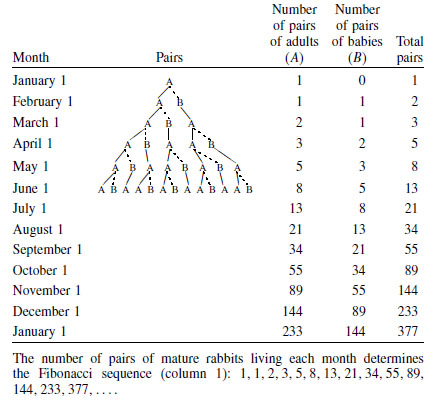If we let fn be the nth term of the Fibonacci sequence, then :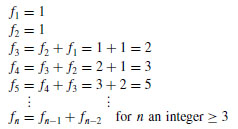That is, each term after the first two terms is the sum of the two preceding terms.

Your students may (rightly) ask at this point, What makes this sequence of numbers so spectacular? For one thing, there is a direct relationship between (believe it or not) it and the Golden Section! Consider successive quotients of the Fibonacci numbers: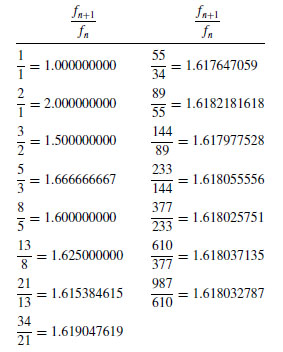Furthermore, you can refer students to notice that successive powers of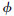* present us with the Fibonacci numbers.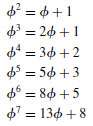If, by now, the students didn’t see the connection, highlight the coefficients and the constants. This is quite incredible; two completely (seemingly) unrelated things suddenly in close relationship to one another. That’s what makes mathematics so wonderful!

* Fibonacci was not a clergyman, as might be expected of early scientists; rather, he was a merchant who traveled extensively throughout the Islamic world and took advantage of reading all he could of the Arabic mathematical writings. He was the first to introduce the Hindu–Arabic numerals to the Christian world in his Liber abaci (1202 and revised in 1228), which first circulated widely in manuscript form and was first published in 1857 as Scritti di Leonardo Pisano (Rome: B. Buoncompagni). The book is a collection of business mathematics, including linear and quadratic equations, square roots and cube roots, and other new topics, seen from the European viewpoint. He begins the book with the comment: “These are the nine figures of the Indians 9 8 7 6 5 4 3 2 1. With these nine figures, and with the symbol 0, which in Arabic is called zephirum, any number can be written, as will be demonstrated below”. From here on, he introduces the decimal position system for the first time in Europe. (Note: The word “zephirum” evolved from the Arabic word as-sifr, which comes from the Sanskrit word, used in India as early as the fifth century,“sunya,” referring to empty.)

## 07 August 2009

### Suprising Number Patterns IV

Here are some more curiosities of mathematics that depend on the surprising nature of its number system. Again, not many words are needed to demonstrate the charm, for it is obvious at ﬁrst sight. Yet in this case, you will notice that much is dependent on the number 1,001, which is the product of 7, 11, and 13. Furthermore, when your students multiply 1,001 by a three-digit number the result is nicely symmetric. For example, 987 • 1,001 = 987,987. Let them try a few of these on their own before proceeding.

Now let us reverse this relationship: Any six-digit number composed of two repeating sequences of three digits is divisible by 7, 11, and 13. For example,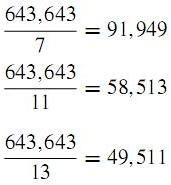We can also draw another conclusion from this interesting number 1,001. That is, a number with six repeating digits is always divisible by 3, 7, 11, and 13. Here is one such example. Have your students verify our conjecture by trying others.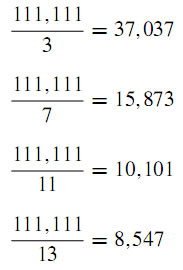What other relationships can be found that play on the symmetric nature of 1,001?

Again, not many words are needed to demonstrate the charm, for it is obvious at ﬁrst sight. These depend on the property described in above and the unusual property of the number 9.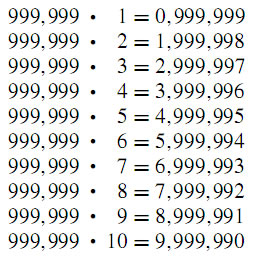Again, the number 9, which owes some of its unique properties to the fact that it is 1 less than the base 10, presents some nice peculiarities.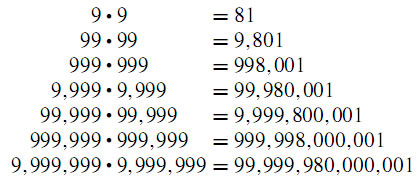While playing with the number 9, you might ask your students to ﬁnd an eight-digit number in which no digit is repeated and which when multiplied by 9 yields a nine-digit number in which no digit is repeated. Here are a few correct choices :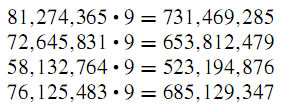Here is another nice pattern to further motivate your students to search on their own for other patterns in mathematics. Again, not many words are needed to demonstrate the beauty of this pattern, for it is obvious at ﬁrst sight.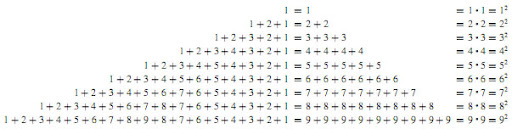## 18 July 2009

### Suprising Number Patterns III

Here are some more charmers of mathematics that depend on the surprising nature of its number system. Again, not many words are needed to demonstrate the charm, for it is obvious at ﬁrst sight. Just look, enjoy, and spread these amazing properties to your students. Let them appreciate the patterns and, if possible, try to look for an "explanation" for this. You might ask them why multiplying by 9 might give such unusual results. Once they see that 9 is one less than the base 10, they might get other ideas to develop multiplication patterns. A clue might be to have them consider multiplying by 11 (one greater than the base) to search for a pattern.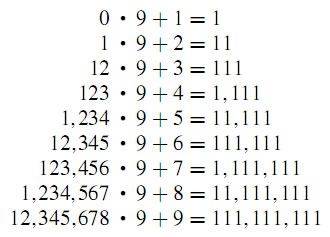A similar process yields another interesting pattern. Might this give your students more impetus to search further?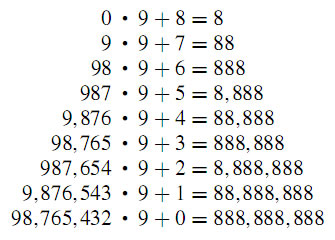Now the logical thing to inspect would be the pattern of these strange products.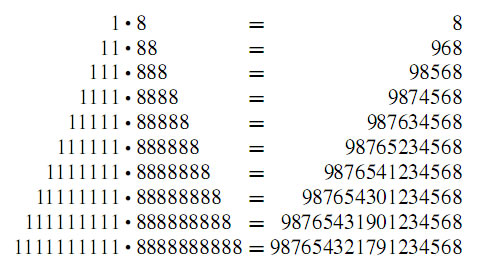How might you describe this pattern? Let students describe it in their own terms.

## 16 July 2009

Google akhirnya merilis SEO starter guide atau panduan dasar SEO dalam 40 bahasa setelah sebelumnya hanya merilis dalam bahasa inggris.

Search Engine Optimization Starter Guide